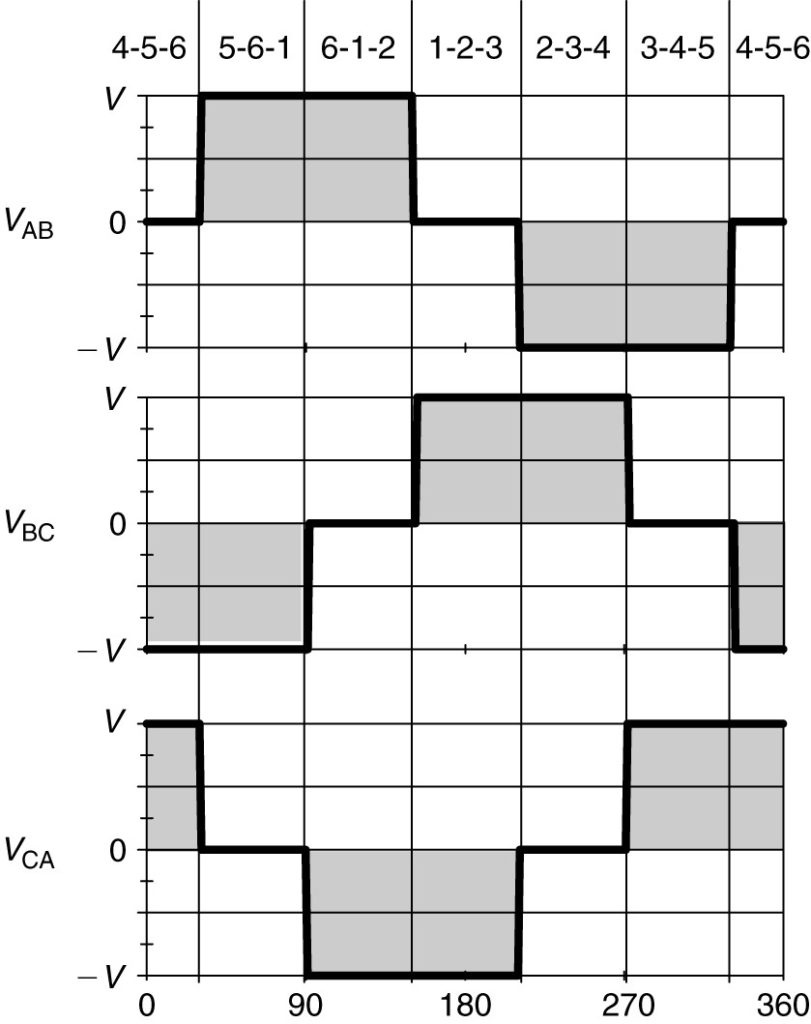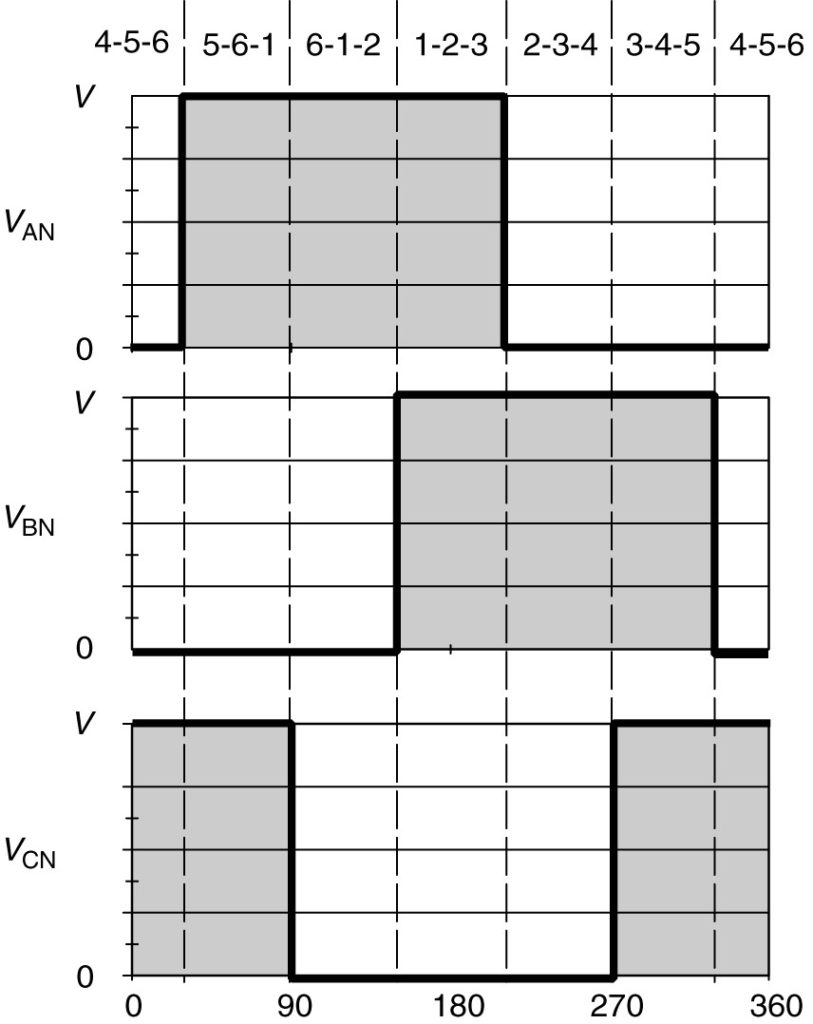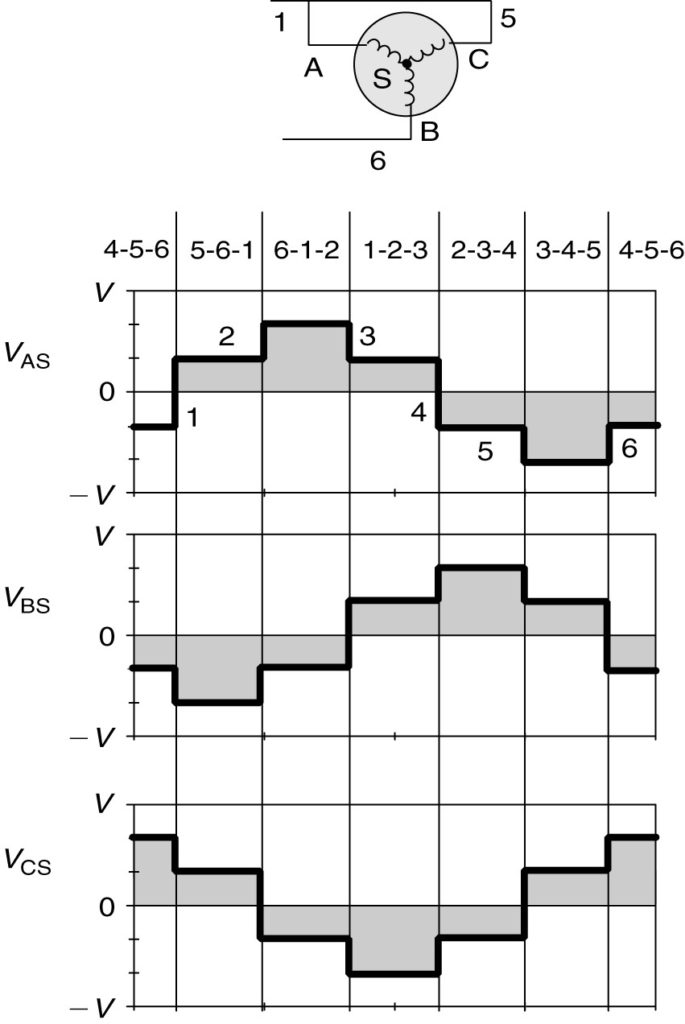Home / DC Machines / Voltage Source Inverters (VSI) Operation | VSI Working Principle

# Voltage Source Inverters (VSI) Operation | VSI Working Principle

Want create site? Find Free WordPress Themes and plugins.

The voltage source inverter is a somewhat older design and less expensive to implement. Various implementations of the VSI are also known as six-step, twelve-step, or even eighteen-step inverters.

## Voltage Source Inverters Operation

In the VSI, the switches are turned on and off at regular intervals to deliver rectangular pulses of voltage to each phase. Figure 1 shows the line-to-line voltages of the inverter, VAB, VBC, and VCA. The line voltages are formed by connecting the line terminals of the motor to either the high or the low side of the DC bus voltage.

Because the three motor terminals must be connected to the two DC terminals, three of the inverter switches will always be closed and, at any point in time, one of the applied line voltages is zero.

The top of Figure 1 shows the pattern of the switching. During the first 30° interval, switches 4, 5, and 6 are closed. Thus, terminals A (switch 4) and B (switch 6) of the motor is connected to the low side of the DC bus and VAB is zero. Switch 5 is in the top half of the bridge and connects terminal C to the high side of the DC bus. Because C is connected to the high side and A to the low side, the line voltage VCA is positive with a magnitude equal to the DC bus voltage. The third line voltage, VBC, is negative, however, because C is at a higher potential than B.

Every 60° one switch turns on while another turns off, resulting in the line voltages shown in Figure 1. Although the voltages are not sinusoidal, they will have a fundamental component and higher harmonics. It can be shown that the rms value of the fundamental is given by

$\begin{matrix} {{V}_{1,rms}}=\frac{\sqrt{6}\text{ }V}{\pi } & {} & \left( 1 \right) \\\end{matrix}$

Where V is the DC bus voltage.FIGURE 1:  Voltage source inverter line voltage waveforms: (a) VAB, (b) VBC, and (c) VCA.

Looking at the switching patterns shown at the top of Figure 1, we can observe that each switch in the bridge remains on for 180°.

For example, switch number 1 turns on at 30° and remains on until 210°, at which point switch 4 turns on, connecting motor terminal A to the low side of the DC bus.

Figure 2 shows the voltages of the motor terminals with respect to the negative terminal of the DC bus. During the time switch 1 is closed, motor terminal A is at the DC voltage, V, while when switch 4 is closed, it is at zero potential with respect to the low side of the DC bus. The voltages at terminals B and C lag the voltage at terminal A by 120° and 240°, respectively.FIGURE 2:  Voltages at motor terminals with respect to the low side of the DC bus.

The phase voltages of the motor are shown in Figure 3. To determine what voltage is actually applied to a phase of the wye-connected motor, consider the small inset in Figure 3, which shows the connections to the motor when switches 5, 6, and 1 are closed. That combination occurs from 30° to 90° on the current waveforms. Since 5 and 1 are closed, phases A and C are connected to the high side of the DC bus. Phase B, on the other hand, is connected to the negative side of the DC bus through switch 6.

The center of the wye- connected windings is called point S in Figures 3. Because phases A and C are connected to the same point, the impedance of those two windings are in parallel between points P and S.

Assuming the two-phase windings have the same impedance, then the parallel combination has one-half the impedance of one winding. Solving the voltage divider formed by the windings, one-third of the DC bus voltage will appear across the parallel A and C windings, while two-thirds appears across the B winding. However, VAS and VCS will be positive, and VBS will be negative. The phase B voltage is negative because the voltage at point S is higher than the voltage at motor terminal B.

Looking at the remaining switching combinations, a phase will always be in series with a parallel combination of the other two phases, or it will be part of a parallel combination that is in series with one phase. As a result, the voltage drop across any given phase will always be plus or minus one-third or two-thirds of the DC bus voltage.FIGURE 3:  Motor line-to-neutral voltages when fed by VSI.

Looking at the phase voltage waveforms in Figure 3, it can be observed that there are six changes in magnitude during one cycle of voltage, as marked on the voltage VAS. Thus, the VSI is frequently called a six-step inverter. Because the waveform is periodic, it contains a fundamental component of voltage as well as higher-order harmonics whose harmonic numbers are given by

$h=6n\pm 1$

where n is an integer from 1 to infinity. Thus, the waveform contains the 5th and 7th, 11th and 13th, 17th and 19th harmonics, and so on.

Other, more complex, circuits are possible that result in 12- or even 18-step waveforms. The advantage of having more steps is a reduction in the lower-order harmonics. For example, a 12- step waveform would have harmonic components numbered

$h=12n\pm 1$

A 12-step inverter would contain harmonics numbered 11 and 13, 23 and 25, 35 and 37, etc. The disadvantage, of course, is that a 12-step inverter costs more than a 6-step inverter since 12 switches and 2 three-phase transformers would be required.

Due to the abrupt changes in voltage in the VSI output, the motor line current tends to be discontinuous and rich in harmonics. A sketch of the typical motor current for a VSI is shown in Figure 4.

At low frequency, the current harmonics create cogging. Cogging means the rotation of the motor shaft is not smooth. This results from the harmonics establishing their own rotating magnetic fields in the motor air gap that create torques, which alternately aid and oppose the rotation of the motor.

The name voltage source inverter actually is something of a misnomer. The inverter can change the frequency of the output waveforms by changing the length of time that the switches are turned on. However, the amplitude of the AC waveform is determined by the DC input voltage. Thus, changing the amplitude of the AC voltage requires a variable DC input to the inverter. This means a controlled rectifier will be required, i.e., SCRs rather than diodes.

One major disadvantage of a controlled rectifier is that the power factor of the drive is a function of the firing angle and can be very low at low frequencies.FIGURE 4:  Motor phase voltage and current when connected to six-step VSI.

Did you find apk for android? You can find new Free Android Games and apps.